# Data Tables in Microsoft Excel

In this article we will learn about how we can create a data table to see the result of multiple inputs at the same time in Microsoft Excel 2013.

Let’s take an example to understand

We’ll build a data table to show the monthly payments for loan terms, with the range of 1 to 6 years, in which number of payments range will be from 12 to 72.

If we use PMT function, we will be able to calculate only the monthly payment for a loan, based on an interest rate, number of periods and the amount of the loan. When we need to adjust the rate, periods or amount, the formula result changes, so we can see the effect of these different variables.

Instead of testing the results with a single formula, we can set up a data table, and compare the results, simultaneously.

We have the following data: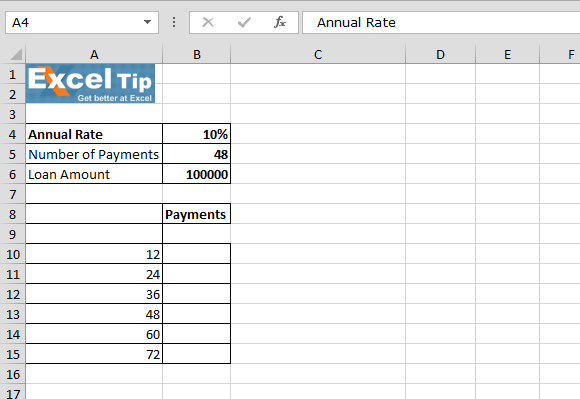First we’ll learn about One variable data table:

• To setup data table we’ll calculate PMT
• Enter PMT function in cell B9
• =PMT(B4/12,B5,B6) [B4 =Annual rate, B5 = Number of payments and B6=Loan Amount]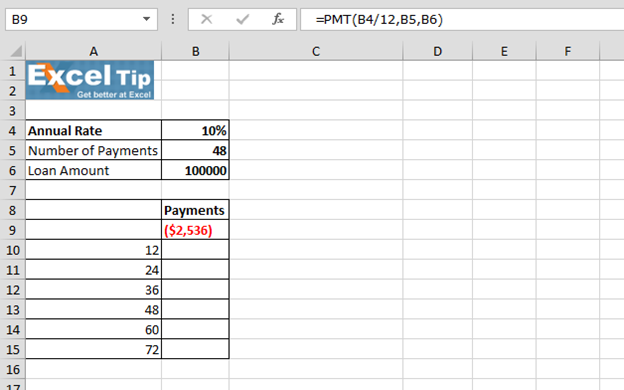• Select cells A9:B15
• In the Data tab > Data tools group > Click on What-if Analysis > Select data table from the list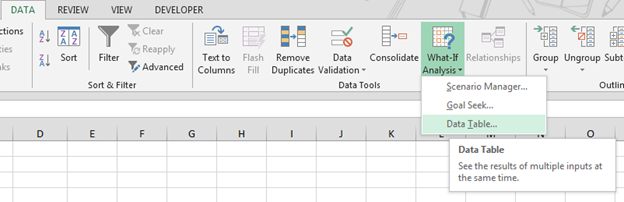• Data table dialog box will appear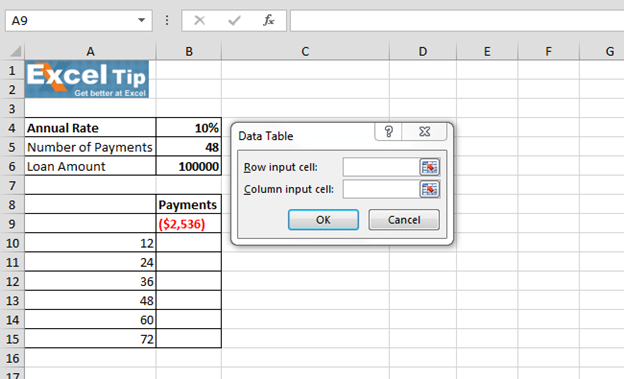• Click in the Column Input cell box, and then click on cell B5, which contains the variable for the number of payments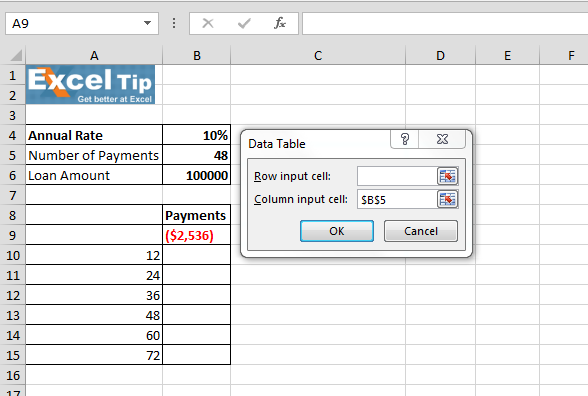• Click OK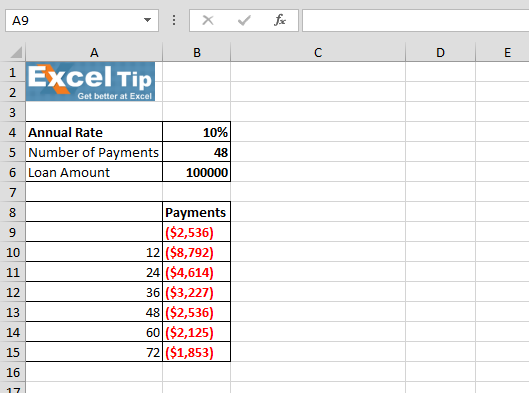• Now select the cells of monthly payments, and format it as Currency. As all the numbers are showing with negative numbers bracketed and in red

Now we’ll clear the data table to create the data table of 2 Variables:

For 2 Variable we have the table below-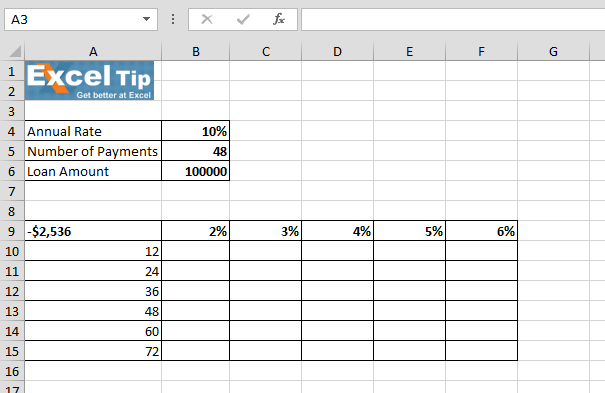• Select the range A9:G15
• Data tab > Data tools group > What-if Analysis drop down list > Data Table
• In Row input cell box we will enter annual rate and in column input cell we will enter number of payments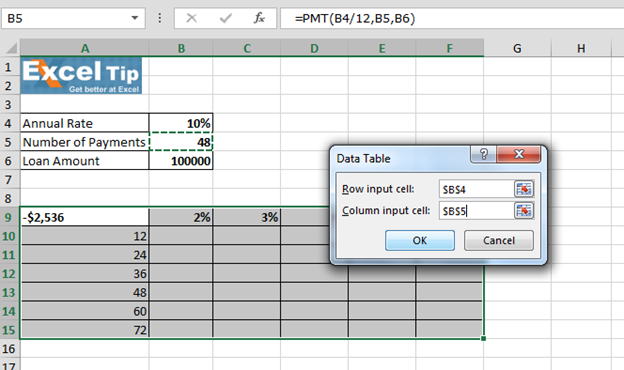• Click OK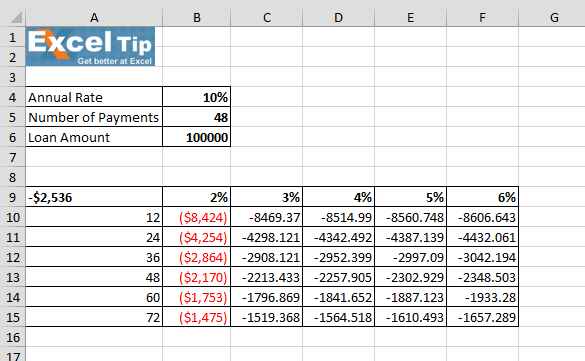When we’ll click on any calculated monthly payments cells and in the formula bar we can see that cell contains TABLE function in which cell B4 is the first argument and cell B5 is the second argument.